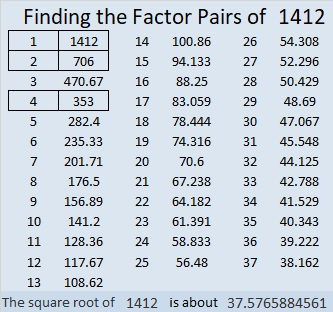# 1412 and Level 3

If you know the greatest common factor of 56 and 48, then you have taken the first step in solving this puzzle. Once you put the factors of 56 and 48 in the appropriate cells, work down from the top of the puzzle to the bottom, cell by cell, until you have put all the numbers from 1 to 10 in both the first column and the top row.Print the puzzles or type the solution in this excel file: 10 Factors 1410-1418

Here are a few facts about the puzzle number, 1412:

• 1412 is a composite number.
• Prime factorization: 1412 = 2 × 2 × 353, which can be written 1412 = 2² × 353.
• 1412 has at least one exponent greater than 1 in its prime factorization so √1412 can be simplified. Taking the factor pair from the factor pair table below with the largest square number factor, we get √1412 = (√4)(√353) = 2√353.
• The exponents in the prime factorization are 2 and 1. Adding one to each exponent and multiplying we get (2 + 1)(1 + 1) = 3 × 2 = 6. Therefore 1412 has exactly 6 factors.
• The factors of 1412 are outlined with their factor pair partners in the graphic below.1412 is the sum of two squares:
34² + 16² = 1412

1412 is the hypotenuse of a Pythagorean triple:
900-1088-1412 calculated from 34² – 16², 2(34)(16), 34² + 16²